Next: Work Up: Conservation of energy Previous: Introduction

## Energy conservation during free-fall

Consider a masswhich is falling vertically under the influence of gravity. We already know how to analyze the motion of such a mass. Let us employ this knowledge to search for an expression for the conserved energy during this process. (N.B., This is clearly an example of a closed system, involving only the mass and the gravitational field.) The physics of free-fall under gravity is summarized by the three equations (24)-(26). Let us examine the last of these equations: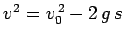. Suppose that the mass falls from height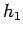to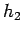, its initial velocity is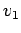, and its final velocity is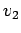. It follows that the net vertical displacement of the mass is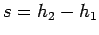. Moreover,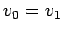and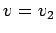. Hence, the previous expression can be rearranged to give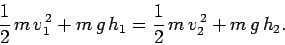(123)

The above equation clearly represents a conservation law, of some description, since the left-hand side only contains quantities evaluated at the initial height, whereas the right-hand side only contains quantities evaluated at the final height. In order to clarify the meaning of Eq. (123), let us define the kinetic energy of the mass,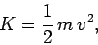(124)

and the gravitational potential energy of the mass,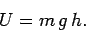(125)

Note that kinetic energy represents energy the mass possesses by virtue of its motion. Likewise, potential energy represents energy the mass possesses by virtue of its position. It follows that Eq. (123) can be written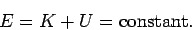(126)

Here,is the total energy of the mass: i.e., the sum of its kinetic and potential energies. It is clear thatis a conserved quantity: i.e., although the kinetic and potential energies of the mass vary as it falls, its total energy remains the same.

Incidentally, the expressions (124) and (125) for kinetic and gravitational potential energy, respectively, are quite general, and do not just apply to free-fall under gravity. The mks unit of energy is called the joule (symbol J). In fact, 1 joule is equivalent to 1 kilogram meter-squared per second-squared, or 1 newton-meter. Note that all forms of energy are measured in the same units (otherwise the idea of energy conservation would make no sense).

One of the most important lessons which students learn during their studies is that there are generally many different paths to the same result in physics. Now, we have already analyzed free-fall under gravity using Newton's laws of motion. However, it is illuminating to re-examine this problem from the point of view of energy conservation. Suppose that a massis dropped from rest and falls a distance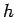. What is the final velocityof the mass? Well, according to Eq. (123), if energy is conserved then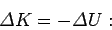(127)

i.e., any increase in the kinetic energy of the mass must be offset by a corresponding decrease in its potential energy. Now, the change in potential energy of the mass is simply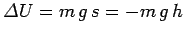, where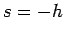is its net vertical displacement. The change in kinetic energy is simply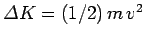, whereis the final velocity. This follows because the initial kinetic energy of the mass is zero (since it is initially at rest). Hence, the above expression yields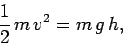(128)

or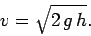(129)

Suppose that the same mass is thrown upwards with initial velocity. What is the maximum heightto which it rises? Well, it is clear from Eq. (125) that as the mass rises its potential energy increases. It, therefore, follows from energy conservation that its kinetic energy must decrease with height. Note, however, from Eq. (124), that kinetic energy can never be negative (since it is the product of the two positive definite quantities,and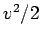). Hence, once the mass has risen to a heightwhich is such that its kinetic energy is reduced to zero it can rise no further, and must, presumably, start to fall. The change in potential energy of the mass in moving from its initial height to its maximum height is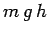. The corresponding change in kinetic energy is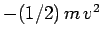; since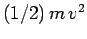is the initial kinetic energy, and the final kinetic energy is zero. It follows from Eq. (127) that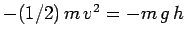, which can be rearranged to give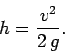(130)

It should be noted that the idea of energy conservation--although extremely useful--is not a replacement for Newton's laws of motion. For instance, in the previous example, there is no way in which we can deduce how long it takes the mass to rise to its maximum height from energy conservation alone--this information can only come from the direct application of Newton's laws.Next: Work Up: Conservation of energy Previous: Introduction
Richard Fitzpatrick 2006-02-02